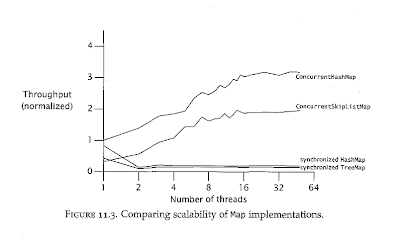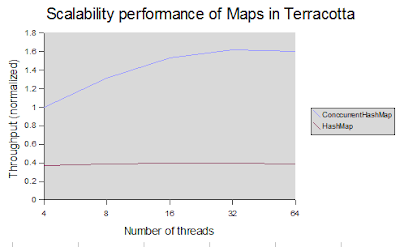## Friday, April 13, 2007

### Performance comparision between ConcurrentHashMap and synchronized HashMap in Terracotta

Since the introduction of ConcurrentHashMap in Java 5, it has been the better choice over HashMap in highly threaded applications. To see how much better, Brian Goetz from his book "Java Concurrency in Practice" wrote a test to compare performance between the two maps. The test sceanario is this:

For N threads concurrently execute a loop that chooses a random key and looks up value corresponding to that key. If the value is found, it is removed with a probability of 0.02. If not, it is added to the map with a probability of 0.6.

The result is ConcurrentHashMap performs much better when number of threads increases. Below is the graph taken from the book with the author's permission.Since Open Terracotta supports ConcurrentHashMap, I wanted so see if the performance advantage is still there (and how much) in distributed environment. My test scenario is modeled after Brian's. However, the number of threads are spread over 4 nodes (Linux RH4). Here is my test code:

`package tc.qa;import java.util.Map;import java.util.Random;import java.util.concurrent.ConcurrentHashMap;import java.util.concurrent.CyclicBarrier;import java.util.concurrent.atomic.AtomicInteger;public class ConcurrentHashMapLoadTest extends Thread {  private static double WRITE_PROBABILITY  = 0.6;  private static double REMOVE_PROBABILITY = 0.02;  private static int    THREAD_COUNT       = 4;  private static int    VM_COUNT           = 4;  private static int    RUNTIME            = 5 * 60 * 1000;  private static int    KEY_RANGE          = 100000;  // roots - in TC world, these are static finals and accessible across JVMs  private Map           map                = new ConcurrentHashMap();  private AtomicInteger throughput         = new AtomicInteger(0);  private CyclicBarrier barrier;  private Random        random;  private int           op;  public ConcurrentHashMapLoadTest() {    random = new Random();    barrier = new CyclicBarrier(THREAD_COUNT);  }  public void run() {    // ready    if (barrier() == 0) {      System.out.println("Started...");    }    // go    long start = System.currentTimeMillis();    while (System.currentTimeMillis() - start < RUNTIME) {      Integer key = new Integer(random.nextInt(KEY_RANGE));      if (get(map, key) != null) {        if (random.nextDouble() < REMOVE_PROBABILITY) {          remove(map, key);          op++;        }      } else {        if (random.nextDouble() < WRITE_PROBABILITY) {          put(map, key, key);          op++;        }      }      op++;    }    throughput.addAndGet(op);    if (barrier() == 0) {      System.out.println("Map type:           "          + map.getClass().getSimpleName());      System.out.println("Runtime:            " + RUNTIME);      System.out.println("Number of threads:  " + THREAD_COUNT);      System.out.println("Write probability:  " + WRITE_PROBABILITY);      System.out.println("Remove probability: " + REMOVE_PROBABILITY);      System.out.println("Ops per second:     "          + (throughput.intValue() * 1000.0 / RUNTIME));    }  }  private int barrier() {    try {      return barrier.await();    } catch (Exception e) {      e.printStackTrace();    }    return -1;  }  private Object get(Map map, Object key) {    if (map instanceof ConcurrentHashMap) {      return map.get(key);    } else {      synchronized (map) {        return map.get(key);      }    }  }  private void put(Map map, Object key, Object value) {    if (map instanceof ConcurrentHashMap) {      map.put(key, value);    } else {      synchronized (map) {        map.put(key, value);      }    }  }  private void remove(Map map, Object key) {    if (map instanceof ConcurrentHashMap) {      map.remove(key);    } else {      synchronized (map) {        map.remove(key);      }    }  }  public static void getParams() {    WRITE_PROBABILITY = Double.parseDouble(System.getProperty("wp", "0.6"));    REMOVE_PROBABILITY = Double.parseDouble(System.getProperty("rmp", "0.02"));    THREAD_COUNT = Integer.parseInt(System.getProperty("thread", "4"));    VM_COUNT = Integer.parseInt(System.getProperty("vm", "4"));    RUNTIME = Integer.parseInt(System.getProperty("runtime", "300000"));  }  public static void main(String[] args) {    getParams();    int threads_per_vm = THREAD_COUNT / VM_COUNT;    for (int t = 0; t < threads_per_vm; t++) {      ConcurrentHashMapLoadTest thread = new ConcurrentHashMapLoadTest();      thread.start();    }  }}`

I wrapped the put(), get() methods to easily switch the map types. After running this test on 4 nodes with jdk1.6.0_01, I got result as below:The Y axis is throughput, normalized to throughput of 4 threads in ConcurrentHashMap.
With network overhead and locks contention in Terracotta, ConcurrentHashMap still far outweights HashMap. Notice how performance of HashMap doesn't degrade much when number of threads increases. This all thanks to the way Terracotta replicates only the delta changes in the map to other nodes. No serialization involved.

The gap between ConcurrentHashMap and HashMap in Terracotta isn't big as it was in the case of 1 JVM like Brian's test. I'm not sure I'm comparing oranges with apples here because obviously it's not the same test, just the same idea. We're always striving for better performance overall and each release has proven that.

Check us out and let us know what you think. http://www.terracotta.org

Hung-